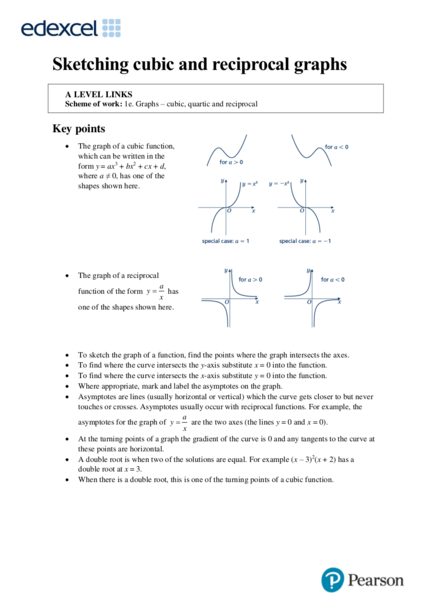# Cubic Graphs and ReciprocalsContributed by:This pdf includes the following topics:-
Sketching cubic and reciprocal graphs
Examples
Practice
Key points
Sketch the graph
1. Sketching cubic and reciprocal graphs
Scheme of work: 1e. Graphs – cubic, quartic and reciprocal
Key points
 The graph of a cubic function,
which can be written in the
form y = ax3 + bx2 + cx + d,
where a ≠ 0, has one of the
shapes shown here.
 The graph of a reciprocal
a
function of the form y  has
x
one of the shapes shown here.
 To sketch the graph of a function, find the points where the graph intersects the axes.
 To find where the curve intersects the y-axis substitute x = 0 into the function.
 To find where the curve intersects the x-axis substitute y = 0 into the function.
 Where appropriate, mark and label the asymptotes on the graph.
 Asymptotes are lines (usually horizontal or vertical) which the curve gets closer to but never
touches or crosses. Asymptotes usually occur with reciprocal functions. For example, the
a
asymptotes for the graph of y  are the two axes (the lines y = 0 and x = 0).
x
 At the turning points of a graph the gradient of the curve is 0 and any tangents to the curve at
these points are horizontal.
 A double root is when two of the solutions are equal. For example (x – 3)2(x + 2) has a
double root at x = 3.
 When there is a double root, this is one of the turning points of a cubic function.
2. Example 1 Sketch the graph of y = (x − 3)(x − 1)(x + 2)
To sketch a cubic curve find intersects with both axes and use the key points above
for the correct shape.
When x = 0, y = (0 − 3)(0 − 1)(0 + 2) 1 Find where the graph intersects the
= (−3) × (−1) × 2 = 6 axes by substituting x = 0 and y = 0.
The graph intersects the y-axis at (0, 6) Make sure you get the coordinates
the right way around, (x, y).
When y = 0, (x − 3)(x − 1)(x + 2) = 0 2 Solve the equation by solving
So x = 3, x = 1 or x = −2 x − 3 = 0, x − 1 = 0 and x + 2 = 0
The graph intersects the x-axis at
(−2, 0), (1, 0) and (3, 0)
3 Sketch the graph.
a = 1 > 0 so the graph has the shape:
Example 2 Sketch the graph of y = (x + 2)2(x − 1)
To sketch a cubic curve find intersects with both axes and use the key points above
for the correct shape.
When x = 0, y = (0 + 2)2(0 − 1) 1 Find where the graph intersects the
= 22 × (−1) = −4 axes by substituting x = 0 and y = 0.
The graph intersects the y-axis at (0, −4)
When y = 0, (x + 2)2(x − 1) = 0 2 Solve the equation by solving
So x = −2 or x =1 x + 2 = 0 and x − 1 = 0
(−2, 0) is a turning point as x = −2 is a
double root.
The graph crosses the x-axis at (1, 0)
3 a = 1 > 0 so the graph has the shape:
3. 1 Here are six equations.
Hint
5
A y B y = x + 3x – 10
2
C 3
y = x + 3x 2
x Find where each
D y = 1 – 3x2 – x3 E y = x – 3x – 1
3 2
F x+y=5 of the cubic
equations cross
the y-axis.
Here are six graphs.
i ii iii
iv v vi
a Match each graph to its equation.
b Copy the graphs ii, iv and vi and draw the tangent and normal each at point P.
Sketch the following graphs
2 y = 2x3 3 y = x(x – 2)(x + 2)
4 y = (x + 1)(x + 4)(x – 3) 5 y = (x + 1)(x – 2)(1 – x)
6 y = (x – 3)2(x + 1) 7 y = (x – 1)2(x – 2)
3 2
8 y= Hint: Look at the shape of y =
a 9 y= 
x x x
in the second key point.
1 1
10 Sketch the graph of y  11 Sketch the graph of y 
x2 x 1
4. 1 a i–C
ii – E
iii – B
iv – A
v–F
vi – D
b ii iv
vi
2 3
4 5
5. 6 7
8 9 y
O x
10 11